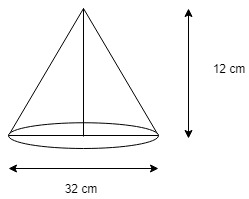# | | Question 44

The height of a conical tank is 12cm and the diameter of its base is 32cm. The cost of painting if from outside at the rate of Rs 21 per sq. m. is
(A) Rs 21210
(B) Rs 11120
(C) Rs 22220
(D) Rs 21120

Explanation:We have to find slant height(l) of the cone.
Radius of cone = 32/2 = 16 cm
l = √(r2 + h2)
l = √(162 + 122)
l = √400 = 20 cm
Cost of painting = Surface area of cone x 21
= πrl x 21
= 22/7 x 16 x 20 x 21
= 21120
Hence, the cost of painting is Rs 21120.

Quiz of this Question
Please comment below if you find anything wrong in the above post

My Personal Notes arrow_drop_up
Article Tags :
Practice Tags :

Be the First to upvote.

Please write to us at contribute@geeksforgeeks.org to report any issue with the above content.